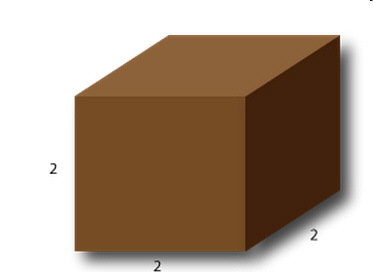6.G.A.2 - Volume
 Name:    6.G.A.2 - Volume

 CompletionComplete each statement. 1. Find the volume of the following diagram:2. Find the Volume of the following: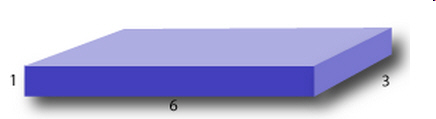3. Find the Volume of the following: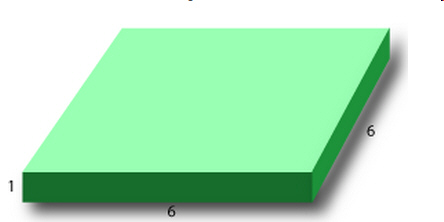4. Find the Volume of the following:5. Find the Volume of the following:6. Find the Volume of the following: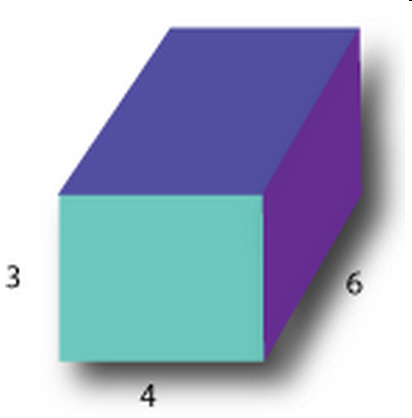7. Find the Volume of the following:8. Find the Volume of the following: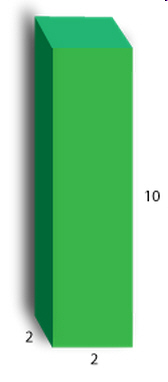9. Find the Volume of the following:10. Find the Volume of the following: